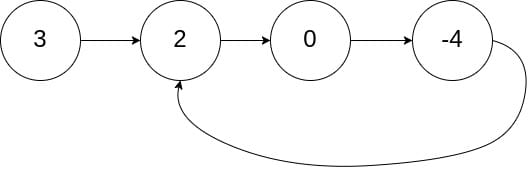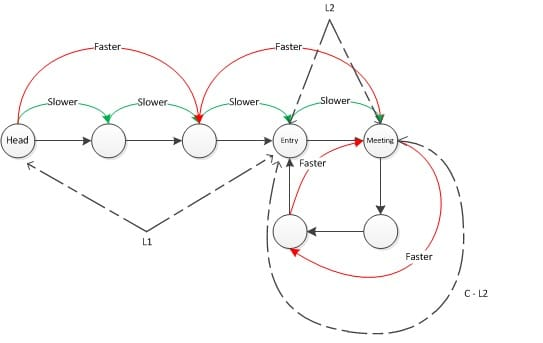# 142. Linked List Cycle II

Reference: LeetCode EPI 7.3
Difficulty: Medium

## Problem

Given a linked list, return the node where the cycle begins. If there is no cycle, return null.

To represent a cycle in the given linked list, we use an integer pos which represents the position (0-indexed) in the linked list where tail connects to. If pos is -1, then there is no cycle in the linked list.

Example:Note: Do not modify the linked list.

Follow up: Cau you solve it without using extra space? $O(1)$

## Analysis

### Hash Set

Time: $O(N)$
Space: $O(N)$ ❌

### Mark Node

It modifies the linked list. ❌

Time: $O(N)$
Space: $O(1)$

### Two Pointers

Why could slow and fast meet? See my analysis in 141. Linked List Cycle

Notations:

• head: the head node of the list.
• entry: the entry node of the cycle.
• meeting: the intersected node at which slow and fast meet.
• L1: the distance between head and entry.
• L2: the distance between entry and meeting.
• C: the length of the cycle.
• n: how many times fast loops in the cycle.Floyd’s Cycle Detection:

The first half is the same as in the two-pointer solution in Problem I. To find the entry point, we can start traversing from head and slow at the meeting point in tandem. The node they meet is the entry node.

Why is the node they meet the entry node?

When slow and fast meet,

• slow moves L1 + L2. (head -> meeting)
• fast moves L1 + L2 + n * C.

Since each time fast moves twice the step as slow does, when they meet at meeting node:

• We have: 2 * Dis(slow) = 2 * (L1 + L2) = L1 + L2 + n * C = Dis(fast) => L1 + L2 = n * C.
• Equivalent to: L1 = (n - 1) * C + (C - L2) where C - L2 is the distance between meeting and entry.
• The equation indicates that if slow or fast now starts moving from meeting and a new node starts moving from head, they will finally meet at the entry node!
• Consider a very big L1 and a relatively short C.
• Note that (n - 1) * C means that slow or fast moves from meeting by n - 1 cycles and still stops at meeting.

Note:

• It’d better to start slow and fast from the head both.
• Move first! Otherwise, they must meet at the beginning.

Time: $O(L1 + L2 + L1) < O(N + N + N) = O(3N) = O(N)$

• Distance that slow moves before they meet: $O(L1 + L2)$
• Distance that the new node moves after slow and fast meet: $O(L1)$

Space: $O(1)$

CommentJunhao Wang
a software engineering cat